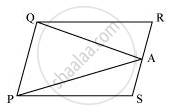# A farmer was having a field in the form of a parallelogram PQRS. She took any point A on RS and joined it to points P and Q. In how many parts the field is divided? What are the shapes of these parts? The farmer wants to sow wheat and pulses in equal portions of the field separately. How should she do it? - Mathematics

A farmer was having a field in the form of a parallelogram PQRS. She took any point A on RS and joined it to points P and Q. In how many parts the field is divided? What are the shapes of these parts? The farmer wants to sow wheat and pulses in equal portions of the field separately. How should she do it?

#### SolutionFrom the figure, it can be observed that point A divides the field into three parts. These parts are triangular in shape − ΔPSA, ΔPAQ, and ΔQRA

Area of ΔPSA + Area of ΔPAQ + Area of ΔQRA = Area of PQRS ... (1)

We know that if a parallelogram and a triangle are on the same base and between the same parallels, then the area of the triangle is half the area of the parallelogram.

∴ Area (ΔPAQ) = 1/2Area (PQRS) ... (2)

From equations (1) and (2), we obtain

Area (ΔPSA) + Area (ΔQRA) = 1/2Area (PQRS) ... (3)

Clearly, it can be observed that the farmer must sow wheat in triangular part PAQ and pulses in other two triangular parts PSA and QRA or wheat in triangular parts PSA and QRA and pulses in triangular parts PAQ.

Concept: Theorem: Parallelograms on the Same Base and Between the Same Parallels.
Is there an error in this question or solution?

#### APPEARS IN

NCERT Class 9 Maths
Chapter 9 Areas of Parallelograms and Triangles
Exercise 9.2 | Q 6 | Page 159